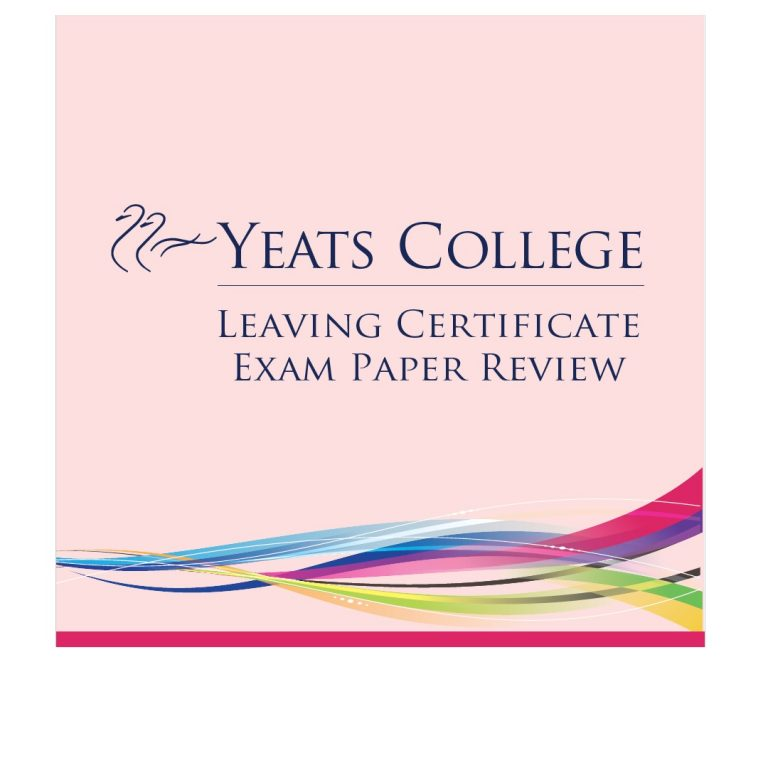# Exam Paper Review – Maths Higher & Ordinary levels

Exam Review – Maths Higher Level Paper 1 – Aisling Kenny Naughton

This afternoon’s Maths paper 1 was a very fair and straightforward exam. Students benefited
from a lot of choice in this year’s adapted paper. They had plenty of time to answer the
required number of questions. There was certainly an emphasis on functions and calculus
throughout the paper. There were, however, some tricky parts to questions that would have
challenged some students.
Section A consisted of six questions and students were required to answer four. The questions
included complex numbers, algebra, functions and calculus. Question 4 involved both a proof
by induction and sequences and series.
Students were required to answer two of the four options in Section B. Question 7 was a
sequences and series question and question 8 involved a cubic function. Question 9 used an
exponential function to model the temperature of coffee as it cools. Students should have
been well prepared for this type of question. Question 10 involved two parts and as a result
was avoided by many. Firstly, students were given a quadratic function for the volume of
water in a tank. They had to answer 4 questions based on that function. Part (b) involved a
trigonometric function representing the increase in the radius of tree trunks.
Overall, students were very happy with this paper. Some were disappointed with the absence
of financial maths. However, those who had worked consistently were rewarded with a
challenging but comprehensive paper.

Review of Mathematics Paper 1 (Ordinary Level) – by Tomás Keane

“Having choice will optimise the chance of success for ordinary level students”

Most students would have found Maths Paper 1 to be a fair and approachable exam. Thankfully, the majority of questions provided little twists and the well-prepared student would have excelled as many questions had appeared in recent years.

Section A provided a lot of scope and options for students, allowing them to cherry pick their favourite topics. This was the first time since the introduction of the Project Maths syllabus that choice was on the paper. One of the main talking points before the exam was whether topics would crossover with one another given the amount of option on the paper. The majority of this exam did not, providing a sigh of relief.

Section A changed this year from being a 25-mark question to a 30-mark question. Although more marks were given, the overall design of the questions didn’t change, thus giving students even more time to answer the paper. In my view, the majority of students would have had plenty of time at the end to answer more questions and to also check their previous work. Very few surprises or twists featured in Section A, which means that students are likely to score quite well.

Section B was also interesting since they added a fourth question. Students were asked to answer two out of four questions, which meant that they could omit questions that were overtly descriptive and instead rely on popular sequencing and graph questions. The main surprise here was the omission of Calculus in the Graphing question. This omission would have favoured the weaker student. Overall, students had their choice between a Financial Maths question, a Volume/Graphing question, a Patterns question, and finally an Area/Motion question. The final question, although manageable, would have been a surprise for most students.

I am very happy with the layout of the paper because it gave students the opportunity to show their knowledge and display their best work. All eyes will now fall to the Paper 2 exam and whether that exam will be more testing of students’ knowledge and skill.  Use the weekend wisely and prepare for popular questions involving The Line, The Circle, Trigonometry, Probability, Statistics, Geometry, Area and Volume.

## Wait!